Bitcoin Forum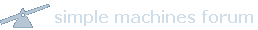September 30, 2020, 02:50:12 PM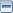News: Latest Bitcoin Core release: 0.20.0 [Torrent]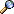Home Help Search Login Register More
 Pages:Author Topic: How to get uncompressed public key from compressed one ?  (Read 3317 times)
Farghaly
Newbie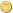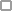Offline

Activity: 38
Merit: 0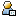June 08, 2014, 11:48:14 PM

Uncompressed public key is:
0x04 + x-coordinate + y-coordinate

Compressed public key is:
0x02 + x-coordinate if y is even
0x03 + x-coordinate if y is odd

How to use this equation to derive the uncompressed public key

y^2 mod p = (x^3 + 7) mod p16014774121601477412

1601477412
 Report to moderator
Advertised sites are not endorsed by the Bitcoin Forum. They may be unsafe, untrustworthy, or illegal in your jurisdiction. Advertise here.
16014774121601477412

1601477412
 Report to moderator
16014774121601477412

1601477412
 Report to moderator
16014774121601477412

1601477412
 Report to moderator
TimS
Sr. MemberOffline

Activity: 250
Merit: 252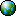June 09, 2014, 12:53:21 AMLast edit: June 09, 2014, 01:14:02 AM by TimSMerited by fronti (1), ETFbitcoin (1)

r^2 = a mod m where m = 3 mod 4 (as secp256k1's p does)
then
r = +-a^((m+1)/4) mod m

So:

y^2 mod p = (x^3 + 7) mod p
y mod p = +-(x^3 + 7)^((p+1)/4) mod p

So calculate (x^3 + 7)^((p+1)/4) mod p, and if the parity of the first answer you get is wrong, then take the negative of that answer (since we're working modulo an odd number, taking the negative will flip the even/odd parity).

Just for fun, here's some Python code that'll do the calculation in the blink of an eye, preset to work on the public key of (the random) private key 55255657523dd1c65a77d3cb53fcd050bf7fc2c11bb0bb6edabdbd41ea51f641

Code:
def pow_mod(x, y, z):
"Calculate (x ** y) % z efficiently."
number = 1
while y:
if y & 1:
number = number * x % z
y >>= 1
x = x * x % z
return number

p = 0xfffffffffffffffffffffffffffffffffffffffffffffffffffffffefffffc2f
compressed_key = '0314fc03b8df87cd7b872996810db8458d61da8448e531569c8517b469a119d267'
y_parity = int(compressed_key[:2]) - 2
x = int(compressed_key[2:], 16)
a = (pow_mod(x, 3, p) + 7) % p
y = pow_mod(a, (p+1)//4, p)
if y % 2 != y_parity:
y = -y % p
uncompressed_key = '04{:x}{:x}'.format(x, y)
print(uncompressed_key)deepceleron
LegendaryOffline

Activity: 1512
Merit: 1001June 09, 2014, 06:07:36 PM

Be mindful that there is no practical reason to "convert" between uncompressed and compressed public keys. Each has their own Bitcoin address - you cannot spend funds sent to the uncompressed public key's address with the compressed public key.ftc-c
NewbieOffline

Activity: 31
Merit: 0May 09, 2015, 03:05:01 PM

Who can give a C++ implementation ?fbueller
Sr. MemberOffline

Activity: 412
Merit: 250May 10, 2015, 01:01:24 AM

@ftc-c: Use libsecp256k1, AFAIK it has preprocessor stuff for C++ support, and it's easy enough to work through.Bitwasp Developer.
samson
LegendaryOffline

Activity: 1778
Merit: 1018May 10, 2015, 05:22:53 PMLast edit: May 10, 2015, 06:58:01 PM by samson

The following link shows how to do it in C using the polarssl / mbedTLS library, you should be able to port this across a different crypto library so long as it has a proper 'bignum'/mpi implementation.

https://gist.github.com/flying-fury/6bc42c8bb60e5ea26631

The above example contains test data as well, from the comments '// Set y2 = X^3 + B' and '// Compute square root of y2' you can see where the important part is done.

I used the above to make a dll/so/dylib which does this useful conversion based on the above code.

What deepceleron said also applies, there are two distinct coin addresses which can be created from a single private key depending on whether the public key is 'compressed' or not.

The above conversion is still useful if you use ECC for things like verifying signatures.CIYAM
LegendaryOffline

Activity: 1890
Merit: 1004Ian Knowles - CIYAM Lead DeveloperMay 10, 2015, 05:28:55 PM

Welcome to use the CIYAM code which includes parts of the Bitcoin code as well (but might be easier to follow in terms of the C++ classes as it doesn't involve any Boost stuff).

https://github.com/ciyam/ciyam/blob/master/src/crypto_keys.cppWith CIYAM anyone can create 100% generated C++ web applications in literally minutes.

GPG Public Key | 1ciyam3htJit1feGa26p2wQ4aw6KFTejU
Blockchain Mechanic
Full MemberOffline

Activity: 382
Merit: 100

Developer and ConsultantJanuary 09, 2017, 08:46:38 AM

Be mindful that there is no practical reason to "convert" between uncompressed and compressed public keys. Each has their own Bitcoin address - you cannot spend funds sent to the uncompressed public key's address with the compressed public key.

Hi

So I could use both addresses to receive funds so long as i have the one private key?Equality vs Equity...
Discord :- BlockMechanic#8560
ranochigo
LegendaryOffline

Activity: 2142
Merit: 1484

@ me if you need my responseJanuary 09, 2017, 01:22:30 PM

Be mindful that there is no practical reason to "convert" between uncompressed and compressed public keys. Each has their own Bitcoin address - you cannot spend funds sent to the uncompressed public key's address with the compressed public key.

Hi

So I could use both addresses to receive funds so long as i have the one private key?

Yes. The compressed public key and the uncompressed ones have different public keys but they can be derived from the same private key. However, they may be different in the Wallet Import Format, private keys starting with 5 are compressed while the uncompressed ones starts from K or L.░░░░░▄▄██████▄▄░░▄████▀▀▀▀▀▀████▄░███▀░░░░░░░░░░▀█▀████░░░▄██████▄▄░░░██░░░░░█████████░░░░██▌░░░░█████████████████░░░░█████████████████░░░░░███████████████████▄░░▀██████▀░░░████▀█▄▄░░░░░░░░░░▄███░░▀████▄▄▄▄▄▄████▀░░░░░▀▀██████▀▀ .ChipMixer.{ MIXING REINVENTED FOR YOUR PRIVACY #.ChipMixer. ░░░░░▄▄██████▄▄░░▄████▀▀▀▀▀▀████▄░███▀░░░░░░░░░░▀█▀████░░░▄██████▄▄░░░██░░░░░█████████░░░░██▌░░░░█████████████████░░░░█████████████████░░░░░███████████████████▄░░▀██████▀░░░████▀█▄▄░░░░░░░░░░▄███░░▀████▄▄▄▄▄▄████▀░░░░░▀▀██████▀▀
chutium
NewbieOffline

Activity: 1
Merit: 0November 26, 2018, 10:41:57 AMLast edit: November 26, 2018, 11:38:56 AM by chutium

I wrote a converter in javascript language, may will be useful for mobile apps or browser extensions.

It is using javascript big integer library latest version 1.6.36, and work with hex string directly:

Code:
const bigInt = require("big-integer");

var retval = '' + number;
while (retval.length < length) {
retval = '0' + retval;
}
return retval;
}

// Consts for secp256k1 curve. Adjust accordingly
// https://en.bitcoin.it/wiki/Secp256k1
const prime = new bigInt('fffffffffffffffffffffffffffffffffffffffffffffffffffffffefffffc2f', 16),

/**
* Point decompress secp256k1 curve
* @param {string} Compressed representation in hex string
* @return {string} Uncompressed representation in hex string
*/
function ECPointDecompress( comp ) {
var signY = new Number(comp) - 2;
var x = new bigInt(comp.substring(2), 16);
// y mod p = +-(x^3 + 7)^((p+1)/4) mod p
var y = x.modPow(3, prime).add(7).mod(prime).modPow( pIdent, prime );
// If the parity doesn't match it's the *other* root
if( y.mod(2).toJSNumber() !== signY ) {
// y = prime - y
y = prime.subtract( y );
}
}

Test with private key from Tim's example 55255657523dd1c65a77d3cb53fcd050bf7fc2c11bb0bb6edabdbd41ea51f641:

Code:
ECPointDecompress('0314fc03b8df87cd7b872996810db8458d61da8448e531569c8517b469a119d267')
returns:
Code:
'0414fc03b8df87cd7b872996810db8458d61da8448e531569c8517b469a119d267be5645686309c6e6736dbd93940707cc9143d3cf29f1b877ff340e2cb2d259cf'

refer to https://stackoverflow.com/a/53480175/5630352

r^2 = a mod m where m = 3 mod 4 (as secp256k1's p does)
then
r = +-a^((m+1)/4) mod m

So:

y^2 mod p = (x^3 + 7) mod p
y mod p = +-(x^3 + 7)^((p+1)/4) mod p

So calculate (x^3 + 7)^((p+1)/4) mod p, and if the parity of the first answer you get is wrong, then take the negative of that answer (since we're working modulo an odd number, taking the negative will flip the even/odd parity).

Just for fun, here's some Python code that'll do the calculation in the blink of an eye, preset to work on the public key of (the random) private key 55255657523dd1c65a77d3cb53fcd050bf7fc2c11bb0bb6edabdbd41ea51f641

Code:
def pow_mod(x, y, z):
"Calculate (x ** y) % z efficiently."
number = 1
while y:
if y & 1:
number = number * x % z
y >>= 1
x = x * x % z
return number

p = 0xfffffffffffffffffffffffffffffffffffffffffffffffffffffffefffffc2f
compressed_key = '0314fc03b8df87cd7b872996810db8458d61da8448e531569c8517b469a119d267'
y_parity = int(compressed_key[:2]) - 2
x = int(compressed_key[2:], 16)
a = (pow_mod(x, 3, p) + 7) % p
y = pow_mod(a, (p+1)//4, p)
if y % 2 != y_parity:
y = -y % p
uncompressed_key = '04{:x}{:x}'.format(x, y)
print(uncompressed_key)Frodek
MemberOffline

Activity: 133
Merit: 18October 07, 2019, 06:02:35 PM

We have:
const prime = new bigInt('fffffffffffffffffffffffffffffffffffffffffffffffffffffffefffffc2f', 16),
if( y.mod(2).toJSNumber() !== signY ) {
// y = prime - y
y = prime.subtract( y );

I wonder, how to compute y = prime - y when y will larger than prime?
(may be problem with signed/big unsinged)
Very large case, I don't know how to reproduce,
this cases will throw away when generated private keys?pooya87
LegendaryOffline

Activity: 2128
Merit: 3134Remember tonight for it's the beginning of foreverOctober 08, 2019, 03:29:14 AM

I wonder, how to compute y = prime - y when y will larger than prime?

in modular arithmetic, the numbers in the group defined by p aren't bigger than p, if they were their remainder (mod p) is calculated hence the finite group. if prime was 5 the group is like this:
{0, 1, 2, 3, 4} when we have 5 we calculate its mod prime and put 0 in its place. for 6 we put 1,...

as for y, it is the vertical coordinate of the points on the curve. and -y is not like regular arithmetic where you flip the sign, -y is defined as (prime - y) so that we mirror the point over x axis and find the other point on curve.░░░░░▄▄██████▄▄░░▄████▀▀▀▀▀▀████▄░███▀░░░░░░░░░░▀█▀████░░░▄██████▄▄░░░██░░░░░█████████░░░░██▌░░░░█████████████████░░░░█████████████████░░░░░███████████████████▄░░▀██████▀░░░████▀█▄▄░░░░░░░░░░▄███░░▀████▄▄▄▄▄▄████▀░░░░░▀▀██████▀▀ .ChipMixer.{ MIXING REINVENTED FOR YOUR PRIVACY #.ChipMixer. ░░░░░▄▄██████▄▄░░▄████▀▀▀▀▀▀████▄░███▀░░░░░░░░░░▀█▀████░░░▄██████▄▄░░░██░░░░░█████████░░░░██▌░░░░█████████████████░░░░█████████████████░░░░░███████████████████▄░░▀██████▀░░░████▀█▄▄░░░░░░░░░░▄███░░▀████▄▄▄▄▄▄████▀░░░░░▀▀██████▀▀
 Pages: